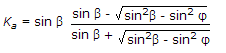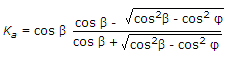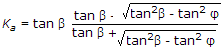# Civil Engineering - Soil Mechanics and Foundation Engineering

Exercise : Soil Mechanics and Foundation Engineering - Section 5
21.
If β is the surcharge angle of a backfill with an angle of internal friction φ, the coefficient of active earth pressure given by Rankine's theory, is :none of these.
Explanation:
No answer description is available. Let's discuss.

22.
A structure is erected on an impervious clay whose thickness is 12 m. Drainage is possible both at upper and lower surfaces. Coefficient of consolidation is 0.015 cm2 per minute. For attaining 50% consolidation with a time factor 0.20, the number of days required
3233
3123
33331
3313
Explanation:
No answer description is available. Let's discuss.

23.
Which one of the following statements is true for Mohr-Coulomb envelope ?
Coulomb suggests that the relationship between shear strength and normal stress, is adequately represented by the straight line
The generalised Mohr theory suggests that, though the shear stress depends on the normal stress, the relation is not linear
Coulomb and Mohr suggest that a definite relationship exists among the principal stress and the angle of internal friction
For an ideal pure friction material, the straight line passes through the origin.
All the above.
Explanation:
No answer description is available. Let's discuss.

24.
The intensity of active earth pressure at a depth of 10 metres in dry cohesionless sand with an angle of internal friction of 30° and with a weight of 1.8 t/m3, is
4 t/m2
5 t/m2
6 t/m2
7 t/m2
8 t/m2